## Voltage Regulation of Transmission Line:

Voltage Regulation of Transmission Line is defined as the rise in voltage at the receiving-end, expressed as percentage of full load voltage, when full load at a specified power factor is thrown off, i.e.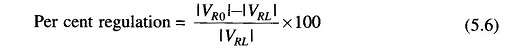where

• |VR0| = magnitude of no load receiving-end voltage
• |VRL| = magnitude of full load receiving-end voltage (at a specified power factor)

For short line, |VR0| = |VS|, |VRL| = |VR|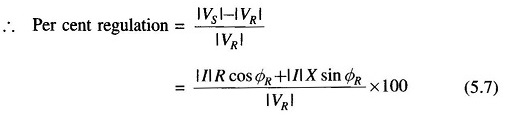In the above derivation, ΦR has been considered positive for a lagging load.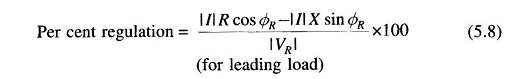Voltage regulation becomes negative (i.e. load voltage is more than no load voltage), when in Eq. (5.8)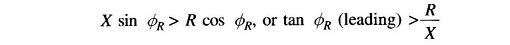It also follows from Eq. (5.8) that for zero voltage regulation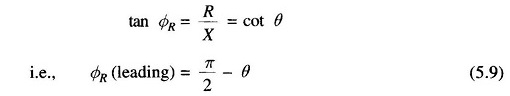where θ is the angle of the transmission line impedance. This is, however, an approximate condition. The exact condition for zero regulation is determined as follows: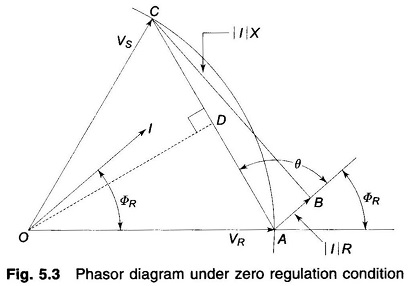Figure 5.3 shows the phasor diagram under conditions of zero voltage regulation, i.e.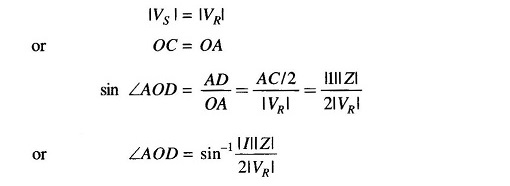It follows from the geometry of angles at A, that for zero voltage regulation,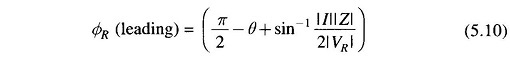From the above discussion it is seen that the voltage regulation of a line is heavily dependent upon load power factor. Voltage regulation improves (decreases) as the power factor of a lagging load is increased and it becomes zero at a leading power factor given by Eq. (5.10).

Scroll to Top